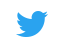# Math HELP!

Developmental Math 1, Developmental Math 2, Core Pre-Algebra and worked examples 1, Brain Teasers, Core Algebra and worked examples 1-4, Core Geometry and worked examples 1, Trigonometry, Probability, Statistics, Precalculus, Calculus, Differential Equations, and Linear Algebra Khan Academy is a vast collection of screencast videos. This site is sponsored by Bill Gates. It will be around for a long time. He is adding more videos daily. The best part is that it is FREE!

WolframAlpha - subjects: Math and Science and much more Need help solving equations? Need all the atomic data on an element? Try this site. You will be impressed. This is the best math website ever. Type in any algebraic equation and it will give the answer and more importantly how to solve it.

Welcome to WebMATH! - Great website that allows you to get help in math on just about any level. NEW****** Math is Fun http://www.mathsisfun.com/index.htm Type in search box.....Provides steps and samples andpractice questions with answers Interactive Mathematics

This is interactive mathematics where you learn math by playing with it! Virtual Manipulatives offer a series of interactive exercises in algebra, geometry, measurements, data analysis and probability.

# Online Math Learning Tools

The resources below are derived from the Mathworks textbooks, Math Explorations.

We have developed interactive math visual models to help students learn the fundamentals of integer addition, subtraction, and multiplication. We have also developed online math flash cards that provide questions aligned to the 6th - 8th grade math TEKS (Texas Essential Knowledge and Skills). We have also provided content for the Personal Financial Literacy TEKS that are relatively new to the math TEKS. Please make use of these resources and share it with other students and teachers!#TWITTER for Math!

Try these twitter hashtags for great math resources (add math terminology or math concept to #hashtag search)

#math or #maths
The most obvious one to begin with, but gives a wide variety of results with added math terminology #hashtags for links to useful maths websites.

#mathchat
Maths teachers exchanging teaching ideas, some of which can be used by parents. Find out more about The Secret Life of #Mathchat

#edutech
Teachers using technology in the classroom and to share teaching ideas. Often there is information here about online conferences for educators.INFOPLEASE